## 3.12.3. Lower-bound and upper-bound markersUp: Derived datatypes Next: Commit and free Previous: Address and extent functions

It is often convenient to define explicitly the lower bound and upper bound of a type map, and override the definition given on page Lower-bound and upper-bound markers . This allows one to define a datatype that has ``holes'' at its beginning or its end, or a datatype with entries that extend above the upper bound or below the lower bound. Examples of such usage are provided in Sec. Examples . Also, the user may want to overide the alignment rules that are used to compute upper bounds and extents. E.g., a C compiler may allow the user to overide default alignment rules for some of the structures within a program. The user has to specify explicitly the bounds of the datatypes that match these structures.

To achieve this, we add two additional ``pseudo-datatypes,'' MPI_LB and MPI_UB, that can be used, respectively, to mark the lower bound or the upper bound of a datatype. These pseudo-datatypes occupy no space (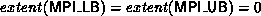). They do not affect the size or count of a datatype, and do not affect the the content of a message created with this datatype. However, they do affect the definition of the extent of a datatype and, therefore, affect the outcome of a replication of this datatype by a datatype constructor.

Example Let D = (-3, 0, 6); T = (MPI_LB, MPI_INT, MPI_UB), and B = (1, 1, 1). Then a call to MPI_TYPE_STRUCT(3, B, D, T, type1) creates a new datatype that has an extent of 9 (from -3 to 5, 5 included), and contains an integer at displacement 0. This is the datatype defined by the sequence {(lb, -3), (int, 0), (ub, 6)} . If this type is replicated twice by a call to MPI_TYPE_CONTIGUOUS(2, type1, type2) then the newly created type can be described by the sequence {(lb, -3), (int, 0), (int,9), (ub, 15)} . (An entry of type ub can be deleted if there is another entry of type ub with a higher displacement; an entry of type lb can be deleted if there is another entry of type lb with a lower displacement.)

In general, if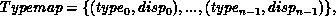then the lower bound of Typemap is defined to be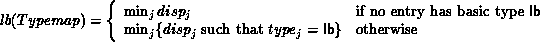Similarly, the upper bound of Typemap is defined to be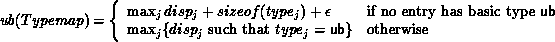Then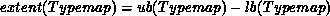If typei requires alignment to a byte address that is a multiple of ki, thenis the least nonnegative increment needed to round extent(Typemap) to the next multiple of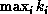.

The formal definitions given for the various datatype constructors apply now, with the amended definition of extent.

The two functions below can be used for finding the lower bound and the upper bound of a datatype.

MPI_TYPE_LB( datatype, displacement)
[ IN datatype] datatype (handle)
[ OUT displacement] displacement of lower bound from origin, in bytes (integer)

int MPI_Type_lb(MPI_Datatype datatype, MPI_Aint* displacement)

MPI_TYPE_LB( DATATYPE, DISPLACEMENT, IERROR)
INTEGER DATATYPE, DISPLACEMENT, IERROR

MPI_TYPE_UB( datatype, displacement)
[ IN datatype] datatype (handle)
[ OUT displacement] displacement of upper bound from origin, in bytes (integer)

int MPI_Type_ub(MPI_Datatype datatype, MPI_Aint* displacement)

MPI_TYPE_UB( DATATYPE, DISPLACEMENT, IERROR)
INTEGER DATATYPE, DISPLACEMENT, IERRORUp: Derived datatypes Next: Commit and free Previous: Address and extent functions